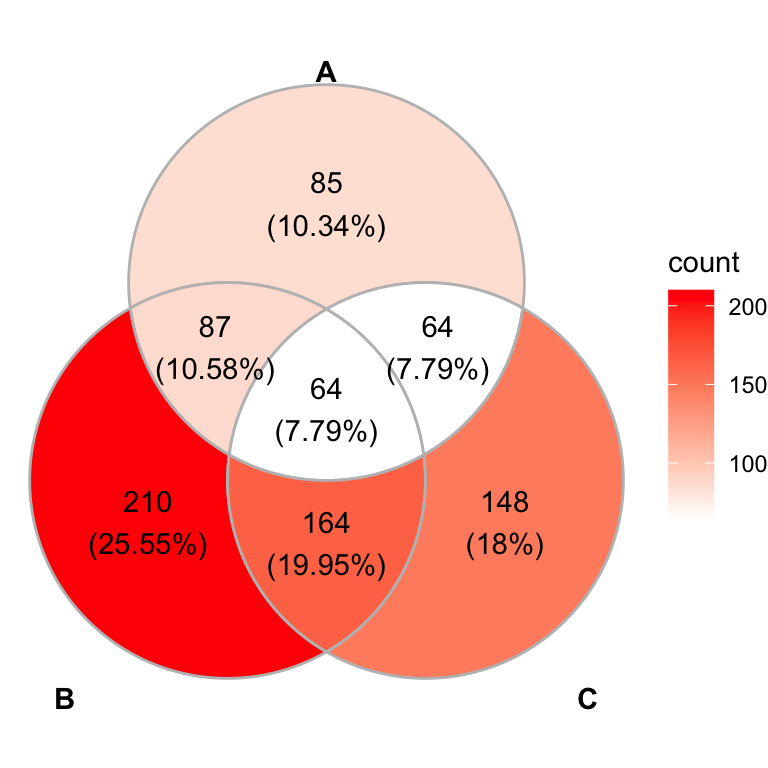# Beautiful GGPlot Venn Diagram with R## Introduction

This article describes how to create a beautiful ggplot Venn diagram. There are multiple extensions of the ggplot2 R package for creating Venn diagram in R, including the ggvenn and the ggVennDiagram packages.

The two packages enable to create Venn plots with 2 to 4 sets or dimensions. The main difference between the two packages is that the ggvenn package assigns a specific color to each set. The ggVennDiagram package maps the fill color of each region to quantity, allowing us to visually observe the differences between different parts.

You will learn how to create Venn diagrams in R using both ggvenn and ggVennDiagram functions.

Contents:

## Create a demo data

set.seed(20190708)
genes <- paste("gene",1:1000,sep="")
x <- list(
A = sample(genes,300),
B = sample(genes,525),
C = sample(genes,440),
D = sample(genes,350)
)

## Create Venn diagrams using the ggVennDiagram R package

### Install and load the ggVennDiagram package

Install the latest development version:

if (!require(devtools)) install.packages("devtools")
devtools::install_github("gaospecial/ggVennDiagram")

library("ggVennDiagram")

### Four dimension Venn plot

library("ggVennDiagram")
# Default plot
ggVennDiagram(x)# Remove labels background color
ggVennDiagram(x, label_alpha = 0)# Change category names
# Change the gradient fill color
ggVennDiagram(
x, label_alpha = 0,
category.names = c("Stage 1","Stage 2","Stage 3", "Stage4")
) +
ggplot2::scale_fill_gradient(low="blue",high = "yellow")### Three dimension Venn plot

ggVennDiagram(x[1:3], label_alpha = 0)### Two dimension Venn plot

ggVennDiagram(x[1:2], label_alpha = 0)## Create Venn diagrams using the ggven R package

### Install and load the ggvenn package

Install the latest development version:

if (!require(devtools)) install.packages("devtools")
devtools::install_github("yanlinlin82/ggvenn")

library("ggvenn")

### Four dimension Venn plot

Note that, the ggvenn() function assigns a specific color to each set.

library("ggvenn")
# Default plot
ggvenn(x)# Change category names
# Change the fill color
names(x) <- c("Stage 1","Stage 2","Stage 3", "Stage4")
ggvenn(
x,
fill_color = c("#0073C2FF", "#EFC000FF", "#868686FF", "#CD534CFF"),
stroke_size = 0.5, set_name_size = 4
)### Three dimension Venn plot

ggvenn(
x, columns = c("Stage 1", "Stage 2", "Stage 3"),
stroke_size = 0.5
)### Two dimension Venn plot

ggvenn(
x, columns = c("Stage 1", "Stage 2"),
stroke_size = 0.5
)## Conclusion

This article describes how to create a ggplot Venn diagram using the ggvernand the ggVennDiagram R packages.

### Comment ( 1 )

•Sewunet Abera

Hello,
Using ggvenn I wanted to list out items in the shared cells.
Example, Stage1 and 2 share 151 items. How can extract these 151 items and track them in my data frame?

Thanks